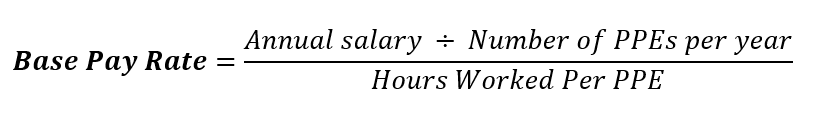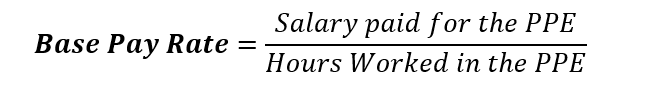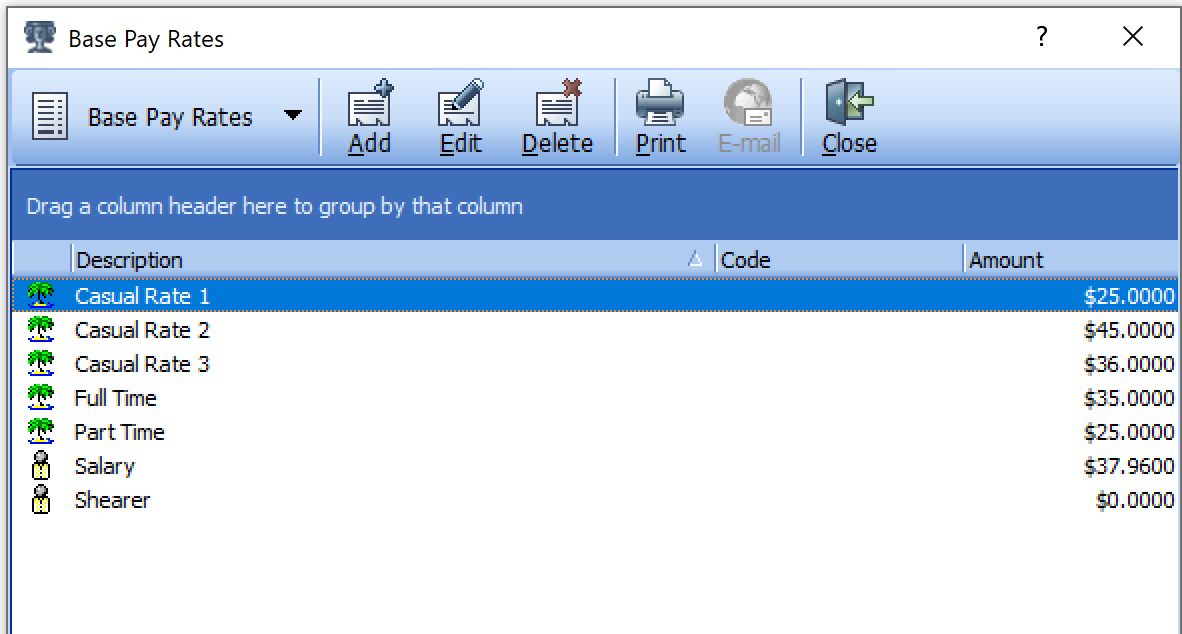Follow

# Calculate Employee Base Pay Rate

Wagemaster has the capacity to recognise ordinary worked hours and calculate any loadings required for alternative worked hours. This ability comes from using the entered timesheet hours, and automatically referencing them against the parameters set down by the employee’s assigned award.

Each employee is attached to a base pay rate in the Employee Details > Employment > Dept/Pay Rate. This base pay rate is the dollar figure applied and paid to each normal hour. It is also the source used in the calculation towards additional payments for alternative hours, like overtime, shift loadings etc. By using a base rate Wagemaster ensures each worked hour is paid to the employee at the correct \$ amount.

To calculate the base pay rate when the Annual Salary and Hours worked are known, use the following formula.To convert an employee’s salaried amount, into an amount they get paid for each hour they work, i.e. base pay rate, please see the formula used to calculate the below examples.• Weekly Salary of \$1,520

Standard Weekly Gross (i.e. \$1,520 per week)

Hours Worked in a Week (i.e. 38)

---------------------------------- = \$ Base Rate (\$40.0000)

• Fortnightly Salary of \$3,040

Standard Fortnightly Salary (i.e. \$3,040)

Hours Worked in a Fortnight (i.e. 76)

-------------------------------------- = \$ Base Rate (\$40.0000)

• Monthly Salary of \$8,233.50

Hours Worked in a Week x 52 Weeks in a year = Hours worked in a Year (i.e. 38 x 52 = 1976)

Hours Worked in a Year (i.e. 1976)

12 Months in a Year

-------------------------------- = Monthly Worked hours (rounded, 164.67)

Standard Monthly Salary (i.e. \$8,233.50)

Monthly Hours Worked (i.e. 164.67)

-------------------------------------- = \$ Base Rate (\$50.0000)

• Annual Salary of \$98,802.00

Hours Worked in a Week x 52 Weeks in a year = Hours worked in a Year (i.e. 38 x 52 = 1976)

Hours Worked in a Year (i.e. 1976)

12 Months in a Year

-------------------------------- = Monthly Worked hours (rounded, 164.67)

Standard Annual Salary (i.e. \$98,802.00)

Annual Hours Worked (i.e. 1976)

-------------------------------------- = \$ Base Rate (\$50.00)

• Annual Salary including Super \$108,188.19

When you are given the annual salary and it includes super, determine what is the salary EXCLUDING super and then calculate the base pay rate: (The super % is 10%).

Annual Salary including super (i.e. \$108,188.19)

Divide by 1.10 (110/100)

-------------------------------- = Annual Salary EXCLUDING super (\$98,352.90)

Hours Worked in a Week x 52 Weeks in a year = Hours worked in a Year (i.e. 38 x 52 = 1976)

Hours Worked in a Year (i.e. 1976)

12 Months in a Year

-------------------------------- = Monthly Worked hours (rounded, 164.67)

Standard Annual Salary (i.e. \$98,802.00)

Annual Hours Worked (i.e. 1976)

-------------------------------------- = \$ Base Rate (\$50.00)

-------------------------------------- = Base Pay Rate (\$24.00)

1. When creating the company database, these base pay rates can be viewed in Setup > Base Pay Rates.2. Please check this list to ensure each employee can be attached to a relevant pay rate.If you are Importing employees or manually entering them through the New Employee Wizard, please be aware that the base pay rate is a mandatory field that must be populated in the process. If there are no base pay rates available, you will be unable to continue with adding the new employee.

Base pay rates can be Added or Edited at any stage.